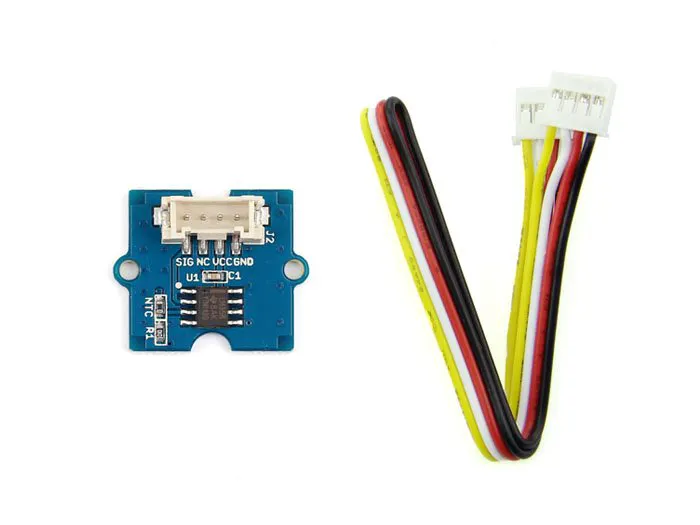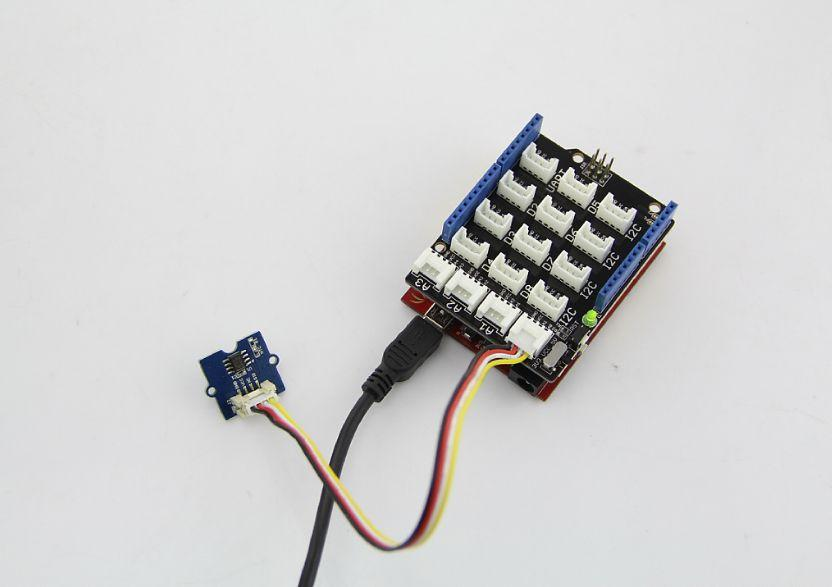# Grove Starter Kit For Arduino --- Temperature Sensor © LGPL

Teaches you how to use the temperature sensor in the Arduino Grove Starter Kit.

• 2,584 views
• 0 comments
• 6 respects

## About this project

Note: I do not talk about the LCD screen in this project, If you haven't yet installed the library for it or need help with it please go here.

### Setup:

As far as hardware, all you need to do is connect the temperature sensor to A0:

### Code:

First you must find the initial value of the thermistor:

``````a = analogRead(0);
``````

Then, since the resistance is what tells us the temperature, we must convert the reading to the resistance:

``````resistance = (float)(1023 - a) * 10000 / a;
``````

And finally we convert the resistance to Celsius:

``````c = 1 / (log(resistance / 10000) / b + 1 / 298.15) - 273.15;
``````

## Code

##### temp.inoArduino
```#include <math.h>
#include <Wire.h>
#include "rgb_lcd.h"
int a; //thermistor value
float c;
int b = 3985;      //b value of the thermistor
float resistance;

const int colorR = 0;
const int colorG = 0;
const int colorB = 255;

rgb_lcd lcd;

void setup(){
lcd.begin(16, 2);
lcd.setRGB(colorR, colorG, colorB);
}

void loop(){
a = analogRead(0); //find the original reading of the thermistor
resistance = (float)(1023 - a) * 10000 / a; //get the resistance of the sensor;
c = 1 / (log(resistance / 10000) / b + 1 / 298.15) - 273.15; //convert to Celsius via datasheet&nbsp;;
int f = (c*(9/5)+32);//convert to F (delete if you want C)
lcd.clear();
lcd.setCursor(0,0);
lcd.print("temperature is:");
lcd.setCursor(0,1);
lcd.print(f);//print the F temp on the lcd screen (change to: lcd.print(c); if you want C)
lcd.print("f");//change to lcd.print("c"); if you want C
delay(1000);//wait 1 second
}
```

## Schematics## Comments

March 11, 2017

#### Members who respect this project

See similar projects
you might like

by Grant

• 1,187 views
• 1 comment
• 2 respects

by Grant

• 3,255 views
• 0 comments
• 9 respects

by Grant

• 11,203 views
• 11 comments
• 10 respects

by Grant

• 8,548 views
• 2 comments
• 10 respects

#### How To Use DS18B20 Water Proof Temperature Sensor

Project showcase by Team IoTBoys

• 89,386 views
• 16 comments
• 26 respects

#### Arduino Temperature Control

Project tutorial by Team pandhoit

• 34,399 views
• 13 comments
• 49 respects